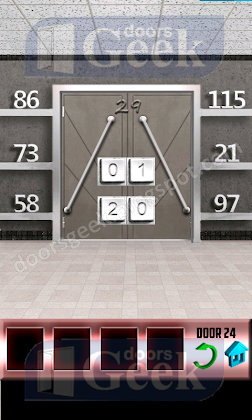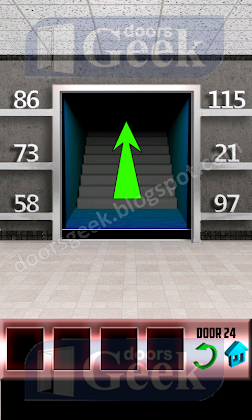## Saturday, April 20, 2013

### 100 Doors Level 24 Walkthrough1. Enter on the top row 21 and on the bottom row 13. But why? The math is this: 86=8+6=14 115=1+1+5=7 14+7= 21(top row) 73=7+3=10 21=2+1=3 10+3=13(bottom row)2. Proceed to the next level.